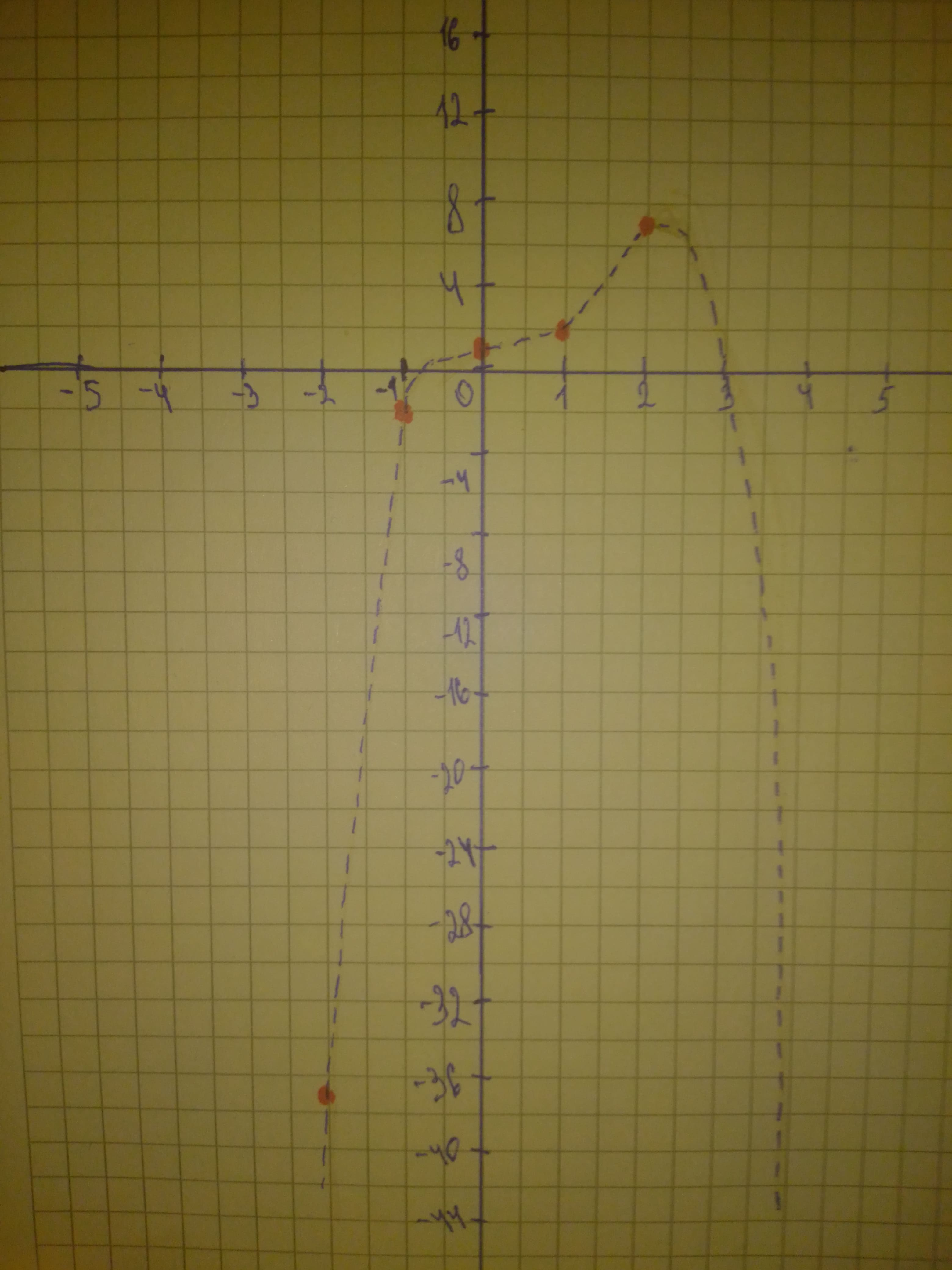# Graph the polynomial function. f(x)=−x^{4}+3x^{3}−x+1slaggingV 2021-02-25 Answered
Graph the polynomial function. $f\left(x\right)=-{x}^{4}+3{x}^{3}-x+1$
You can still ask an expert for help

• Questions are typically answered in as fast as 30 minutes

Solve your problem for the price of one coffee

• Math expert for every subject
• Pay only if we can solve ittabuordy

Step 1
$f\left(x\right)=-{x}^{4}+3{x}^{3}-x+1$
The leading coefficient is -1 (negative) and the degree is 4 (even)
Therefore
and
Step 2